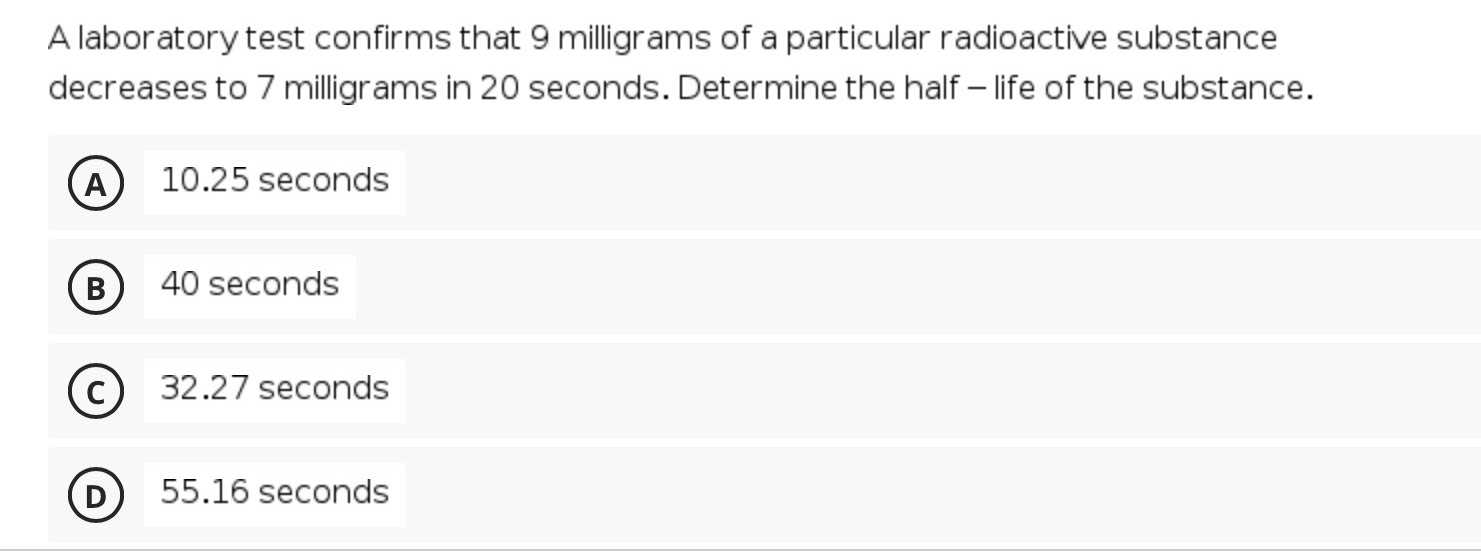### ¿Todavía tienes preguntas de matemáticas?

Pregunte a nuestros tutores expertos
Algebra
PreguntaA laboratory test confirms that $$9$$ milligrams of a particular radioactive substance decreases to $$7$$ milligrams in $$20$$ seconds. Determine the half - life of the substance.

(A) $$10.25$$ seconds (B) $$40$$ seconds (C) $$32.27$$ seconds (D) $$55.16$$ seconds

$$D$$